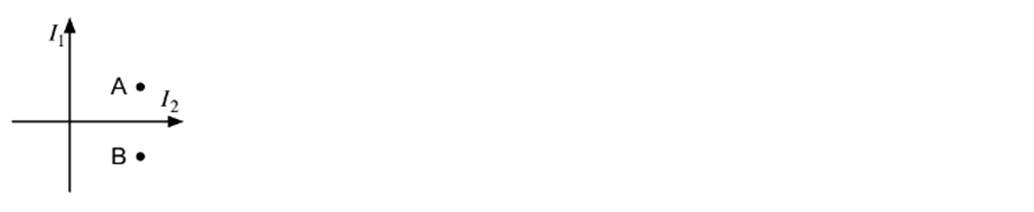# Problem: Two long wires carrying currents I 1 and I2 in the directions shown, with I 2 = 4I1, cross at right angles in the xy plane. Consider the points A = (2d, d) and B = (2d, −d), where d is a positive number. Find the ratio of the magnitudes of the magnetic fields at these two points, i.e., what is |BB/BA|? A) 9/7 B) 1 C) 5/3 D) 4 E) None of these

🤓 Based on our data, we think this question is relevant for Professor Buehrle's class at UMD.

###### Problem Details

Two long wires carrying currents I 1 and I2 in the directions shown, with I 2 = 4I1, cross at right angles in the xy plane. Consider the points A = (2d, d) and B = (2d, −d), where d is a positive number. Find the ratio of the magnitudes of the magnetic fields at these two points, i.e., what is |BB/BA|?

A) 9/7

B) 1

C) 5/3

D) 4

E) None of these